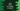# Java program to check if a number is special or not## Java program to check if a number is special or not:

In this post, we will learn how to check if a number is special or not in Java. If the sum of factorials of a number is equal to the number, then it is called special. For example, 145 is a special number. For 145, 1! + 4! + 5! = 1 + 24 + 120 = 145. So, it is a special number.

## Algorithm for the program:

We will use the below algorithm to solve this problem:

• Get the number as input from the user.
• Initialize one sum variable as 0 to hold the final sum of all factorials of the digits.
• Find the factorial of each digits of the number one by one.
• Add the factorials to the final sum digit.
• Compare the sum digit with the input number. If both are equal, it is a special number. Else, it is not.

So, we need to create two methods here. One to check if the number is special or not and the second one to find factorial of a number.

## Java program to check if a number is special:

Below is the complete program:

``````import java.util.Scanner;

class Main {
// 1
private static int findFactorial(int n) {
int fact = 1;
for (int i = 2; i <= n; i++) {
fact *= i;
}
return fact;
}

public static void main(String[] args) {
// 2
int number, lastDigit;
int sum = 0;
Scanner sc = new Scanner(System.in);

// 3
System.out.println("Enter the number: ");
number = sc.nextInt();

// 4
int numberCopy = number;

// 5
while (numberCopy != 0) {
lastDigit = numberCopy % 10;
sum += findFactorial(lastDigit);
numberCopy /= 10;
}

// 6
if (number == sum) {
System.out.println("It is a special number");
} else {
System.out.println("It is not a special number");
}

}
}``````

### Explanation:

The commented numbers in the above program denote the step numbers below:

1. findFactorial is a method to calculate the factorial of a number. It takes one number and returns its factorial value.
2. We have created two integers number and lastDigit to hold the user input number and the last digit of the number. We will keep removing the last digit of the number. sum is to store the sum of the factorials of each digits. sc is a Scanner variable, this is used to read the user input.
3. It is asking the user to enter the number. It reads that value and stores it in the variable number.
4. numberCopy is a variable, to keep a copy of the user input number.
5. Using a while loop, we are getting the last digit of the copied number, calling findFactorial to find the factorial for that digit, adding that to the sum variable and removing the last digit of that number.
6. This step checks if the number is equal to the sum of factorials or not. If yes, it prints that it is a special number, else it prints that it is not a special number.

## Method 2: By using a HashMap:

We can also use a HashMap and keep the factorials of all digits from 0 to 9. We don’t have to recalculate the fatorial of digits again and again. We can simply pick it from the dictionary.

Below is the complete program:

``````import java.util.HashMap;
import java.util.Map;
import java.util.Scanner;

class Main {
public static void main(String[] args) {
int number, lastDigit;
int sum = 0;
Scanner sc = new Scanner(System.in);

Map<Integer, Integer> factorialMap = new HashMap<Integer, Integer>() {{
put(0, 1);
put(1, 1);
put(2, 2);
put(3, 6);
put(4, 24);
put(5, 120);
put(6, 720);
put(7, 5040);
put(8, 40320);
put(9, 362880);
}};

System.out.println("Enter the number: ");
number = sc.nextInt();

int numberCopy = number;

while (numberCopy != 0) {
lastDigit = numberCopy % 10;
sum += factorialMap.get(lastDigit);
numberCopy /= 10;
}

if (number == sum) {
System.out.println("It is a special number");
} else {
System.out.println("It is not a special number");
}

}
}``````

Here,

• Everything is kept same, except we have defined one HashMap factorialMap. The key for the HashMap is 0 to 9 and value is the factorial for each digit. e.g., if we want to find the factorial of 9, we can get the value with key 9 from this dictionary.
• Inside the while loop, when we are calculating the factorial, we are not calling any method to calculate this, we are simply getting this value from the HashMap.

### Output:

If you run any of these programs, it will print output as like below:

``````Enter the number:
145
It is a special number

Enter the number:
1000
It is not a special number``````